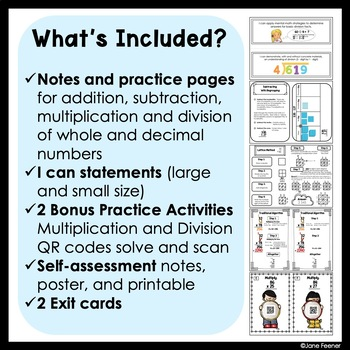# Interactive Math Journal for Operations4th - 5th, Homeschool
Subjects
Standards
Resource Type
Formats Included
• Zip
Pages
204 pages

### Description

This Interactive Math Journal for operations contains notes and foldables to teach your students about addition, subtraction, multiplication and division. These pages can be used to introduce a concept or as an activity to review key points of a math lesson. This Interactive Math Journal will make a great reference tool for students to use throughout the school year. Please check the topics covered below as not all topics pertaining to operations are covered. Also if you bought my Interactive Math Journal for Place Value there a few pages that are the same. For example: the cover pages, table of contents and assessment sheets.

This unit contains a “starter kit” for teaching operations. What’s included:
• Achievement indicators and I can statements for various operation concepts. There is a PowerPoint file, which contains blank cards so they can be edited to meet your individual needs.
• Notes and foldables to complete a double-page spread for each concept or skill. There are pictures of each finished entry to show what the completed pages will look like. (Please check the preview for more details as to what is included in this unit)
• Two QR activities to go with the concepts being taught in the journal. One of the QR codes activities is 2 digit by 2 digit multiplication and division 3 digit by 1 digit.
• An exit card for multiplication and division and a method for student’s self-assessment of their understanding of each concept.

Operation topics covered are:

• Estimation strategies – (Front-end, compatible numbers, and compensation)
• Addition of whole numbers using base ten blocks and place value
• Adding with no regrouping and with regrouping
• Subtraction of whole numbers (no regrouping and with regrouping)
• Addition of decimal numbers (with base ten blocks and place value)
• Subtraction of decimal numbers (with base ten blocks and place value)

Multiplication
• Multiplication strategies
• Adding zeros when one factor is 10, 100, or 1000
• Doubling and halving
• Distributive property

Multiplication
• One digit by 3, and 4 digit numbers
• Multiplication 2 digit by 2 digit
Array (Base ten)
Array (Box method)
Partial Product
Lattice Method
Cluster Method
Flipbook

Division
• Division strategies and divisibility rules
• Sharing using base ten blocks
• Chunking
• Traditional algorithm (using the division family)
• Interpreting remainders
• Checking division using multiplication

Create and solve your own problem.

This is a zipped file that includes: a green covered file which contains all the notes and foldables. The second orange file contains “I Can” statements to meet Common Core Standards 4.NBT.4, 4.NBT.5, 4.NBT.6.

You may also like the following products:

Place Value Interactive Notebook

Fractions Interactive Notebook

Decimal File Lapbook

Any questions or suggestions please email me at janefeener@gmail.com.
Total Pages
204 pages
Included
Teaching Duration
2 months
Report this Resource to TpT
Reported resources will be reviewed by our team. Report this resource to let us know if this resource violates TpT’s content guidelines.

### Standards

to see state-specific standards (only available in the US).
Find whole-number quotients and remainders with up to four-digit dividends and one-digit divisors, using strategies based on place value, the properties of operations, and/or the relationship between multiplication and division. Illustrate and explain the calculation by using equations, rectangular arrays, and/or area models.
Multiply a whole number of up to four digits by a one-digit whole number, and multiply two two-digit numbers, using strategies based on place value and the properties of operations. Illustrate and explain the calculation by using equations, rectangular arrays, and/or area models.
Fluently add and subtract multi-digit whole numbers using the standard algorithm.